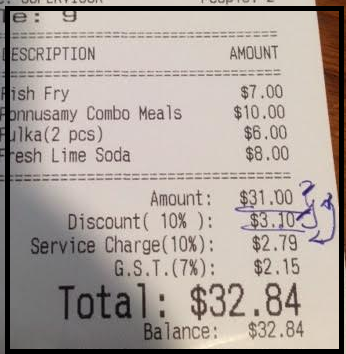# [Solved] How to calculate Service charge on balance amount i-e after bill discounted

i want to set service charge on [(Ticket Amount - Bill Discount)] Amount.

i know i have to set calculation type to custom but how do i deal with it?

i used this in scriipt

result = (Ticket.PSum + Ticket.PreSum - Ticket.Tax) * 0.10

i want the result to be (ticket total - bill discount ) * 0.10

while using this i am not getting the desired calculations

Ticket.Tax is not the same as a discount.

then what syntax is used for bill discount on settle screen

i have tried result = (Ticket.PSum + Ticket.PreSum - Ticket.Discount) * 0.10

but i think there is no such variable Ticket.Discount

PSum is alredy non discounted amount. PreSum is pre tax servicing amount so it is probably discount amount for your case. Now I need to understand why you’re summing these and want to subtract discount back. Did you tried what PSum and PreSum values returns?

i did try this

and i want to calculate service from (Ticket Total - Bill Discount) has been given

What does that returns? Why you’re summing these?

this is what i get

and if i use Ticket.PSum - Ticket.PreSum

i want to calculate service charge from (( ticket total - bill discount) amount ) * 0.10OK. This is better. You don’t need custom calculation for that. Select `Rate from Previous template amount`. Be sure calculations are sorted properly so discount calculates first.

1 Like

@emre Ticket.PSum + Ticket.PreSum is working

i got confused with the total and balance amount

why does it show two different amount

Its working thankyou

but can you explain the diffference between the script Ticket.PSum + Ticket.PreSum and Rate from Previous template amount

Your calculation changes Ticket.PreSum value as it configured as a pre tax service. PreSum is not only discount. It returns total of all services.

1 Like